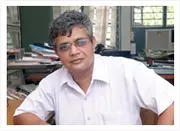X
Investigation of Postflooding Conditions in Countercurrent Gas-Liquid Flow
S. Maharudrayya,
Published in
2002
Volume: 48

Issue: 2
Pages: 212 - 220
Abstract
Systematic experiments were conducted to study the postflooding situation in air - water countercurrent flow in a tube of 0.025 m internal diameter with smooth inlet and outlet conditions for the two phases. The downflow rate of water and the pressure gradient were measured over a range of flow rates for test-section lengths of 0.6, 1.2 and 2.0 m. Additional experiments were conducted with increasing and decreasing water flow rates at a constant air flow rate. The length of the test section or the way the flooding point was approached did not significantly affect the onset of flooding. The data show that, as the gas flow rate is increased, the pressure gradient can fall significantly in the postflooding situation due to depleting the downflow rate of liquid. Dimensionless correlations are proposed to calculate the downflow rate and the pressure gradient given for overall flow parameters.
Journal AIChE Journal 00011541 No
Authors (1)
•Concepts (19)
•Correlation methods
•Pressure effects
•Water
•POSTFLOODING CONDITIONS
•Flow of fluids
•Air
•Flow velocity
•GAS-LIQUID TWO-PHASE FLOW
•Mathematical analysis
•Airflow
•Article
•Calculation
•COUNTERCURRENT SYSTEM
•Flow rate
•Gas flow
•Molecular mechanics
•••